## Friday, October 28, 2016

One skill that students need to master in eighth grade is functions. In sixth and seventh grade students lay the foundations for equations and functions. Now that they are in eighth grade they are using the equation skills from sixth and seventh grade. Students often make mistakes with setting up the function table, making connections between the function table and graph or function table and equation. These are simple mistakes that you can prevent with practice.

I have listed here activities to review and practice Functions. I hope these help jump start your lesson planning. The key to getting your students involved in the learning process is to include interactive, visual, engaging activities for them to actively participate in.This math station activity is intended to help students understand how to graph proportional relationships, understand that a function is a rule that assigns one input to one output exactly, compare properties of two functions, interpret the equation y = mx + b, construct a function, determine the rate of change of a function, and describe the functional relationship between two quantities.

Included are:
-6 different stations to engage students
-Teacher facilitated activity for 60-90 minutes of classroom time
-Stations include:
1. Vocabulary
3. Practice of Functions
5. Real World applications of Functions
6. Word problems of Functions
-Student Station Guide helps students record their answers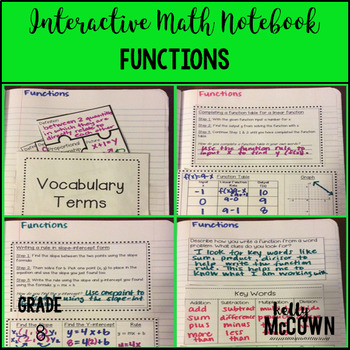These interactive notebook activities are intended to help students understand how to graph proportional relationships, understand that a function is a rule that assigns one input to one output exactly, compare properties of two functions, interpret the equation y = mx + b, construct a function, determine the rate of change of a function, and describe the functional relationship between two quantities.

Included are:
-4 different Functions activities to engage students
-Teacher facilitated activity for 60-90 minutes of classroom time
-Activities include: Vocabulary, Completing a function table and graph from a linear function rule, Writing a rule from two points using slope-intercept form, and Writing a rule, completing a function table, and drawing a graph from a function word problem.
-Examples of Completed Interactive Math Notebook Activities are included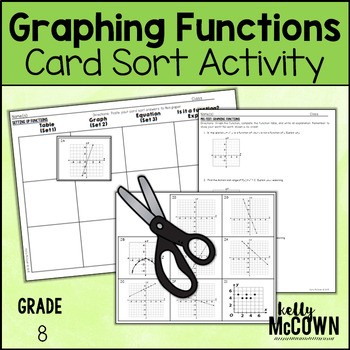This lesson unit is intended to help you assess how well students are able to assess and graph functions in tables, graphs, and equations.

Included in this lesson are:
-card sort activity with concept development of functions
-one extension activity for students to deepen their conception of functions
-answer keys for all the assessments and activities
-how the lesson is tied to Common core and mathematical practices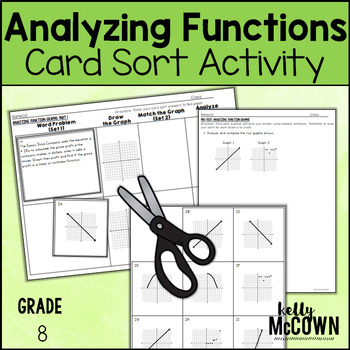This lesson unit is intended to help you assess how well students are able to describe qualitatively the functional relationship between two quantities by analyzing a graph (e.g., where the function is increasing or decreasing, linear or nonlinear). Sketch a graph that exhibits the qualitative features of a function that has been described verbally.

Included in this lesson are:
-card sort activity with concept development of analyzing functions
-one extension activity for students to deepen their conception of analyzing functions
-answer keys for all the assessments and activities
-how the lesson is tied to common core and mathematical practices

I hope these resources are helpful in getting your students engaged in Functions. Keeping students actively engaged in the learning process will ensure that they are in charge of their own learning. The goal of learning Functions is to set up the foundations for Algebra skills in the future. Students must master functions in eighth grade to be able to achieve success in Algebra. Your teaching is important and the students need to actively be engaged in the process.

## Wednesday, October 26, 2016

### Halloween Math Activities {Grades 3 to 8}

Teaching around Halloween can be a tricky time of year. Why? Lots of candy is everywhere! Kids want to celebrate it at school and not everyone celebrates Halloween in their culture. Still want to celebrate it? Read this article about religions and cultures that do not celebrate Halloween and where Halloween came from {Click Here}.

My focus during Halloween has always been on pumpkins and fun engaging FALL activities. There are many cultures and religions that do not allow children to participate in Halloween, so instead of being in a conversation with your principal, plan ahead for SAFE FALL-FRIENDLY Math activities in your classroom.

Here are some of my favorites:

All of these Math activities are for Grades 3, 4, 5, 6, 7, and 8. Fun, engaging, and great review for all students! Teachers have used these packets for extra credit, classwork, homework, math station activities, math center activities, and fast finishers to name a few.

CLICK HERE to check them out at my TPT Store. I also give out FREE activities to my Followers, so if you click "FOLLOW ME" on TPT you will receive some FREE Math activities in November. I know these activities will help engage your students in Math and be lots of fun!

## Monday, October 24, 2016

### Teaching Proportions & Percents {Grade 7}

One skills that is often difficult for seventh grade students is learning proportions and percents. In sixth grade students learn the foundations for how to solve proportions for seventh grade. Now that they are in seventh grade they need to use those foundational skills to build upon. Students often make mistakes with setting up proportions, multiplying and solving proportions, and setting up to solve percents.

I have listed here activities to review and practice Proportions and Percents. I hope these help jump start your lesson planning into the world of Proportional Relationships. The key to getting your students involved in the learning process is to include interactive, visual, engaging activities for them to actively participate in.These interactive notebook activities are intended to help students understand how to use proportional relationships to solve multistep ratio and percent problems, understand that rewriting an expression in different forms in a problem context can shed light on the problem, and solve multi-step real-life and mathematical problems posed with rational numbers.

Included are:
-4 different Proportions & Percents activities to engage students
-Teacher facilitated activity for 60-90 minutes of classroom time
-Activities include: Vocabulary, finding the part, whole, or Percent, Finding Sales tax and Discounts, and Finding Simple Interest.
-Examples of Completed Interactive Math Notebook Activities are includedThis math station activity is intended to help students understand how to use proportional relationships to solve multistep ratio and percent problems, understand that rewriting an expression in different forms in a problem context can shed light on the problem, and solve multi-step real-life and mathematical problems posed with rational numbers.

Included are:
-6 different stations to engage students
-Teacher facilitated activity for 60-90 minutes of classroom time
-Stations include:
1. Vocabulary
3. Practice of Proportions & Percents
4. Additional Practice of Proportions & Percents
5. Real World applications of Proportions & Percents
6. Word problems of Proportions & Percents
-Student Station Guide helps students record their answersThese math foldable notes are for students to be able to understand compute unit rates, ratios with fractions, measure quantities in like or different units, recognize and represent proportional relationships, decide whether two quantities are proportional, identify the constant of proportionality (unit rate), show the unit rate in tables, graphs and equations, represent proportional relationships by equations, explain points on a graph of a proportional relationship, and use proportional relationships to solve multistep ratio and percent problems.

Included are:
-7 different NO PREP Math Foldable Notes to engage students in note taking
-Teacher facilitated activity for 15-20 minutes of classroom time each
-PRINT & GO foldable notes that are ready with NO PREP
-Directions for using Math Foldables in your classroom
-Options for scaffolding with blank templates
-Foldables included:
Foldable: Percent of a Number
Foldable: Percent Proportion
Foldable: Percent and Estimation
Foldable: The Percent Equation
Foldable: Percent of Change
Foldable: Sales Tax and Discount
Foldable: Simple Interest
Foldable: Four Blank Templates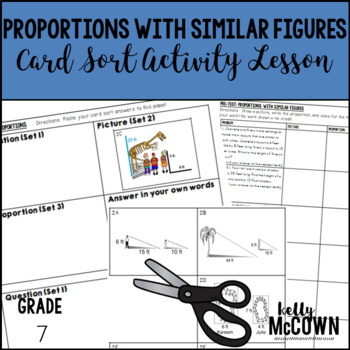This lesson unit is intended to help you assess how well students are able to identify when two quantities vary in direct proportion to each other, represent two quantities as directly proportional relationships, and solve proportional problems using efficient methods.Included in this lesson are:
-card sort activity with concept development of proportions
-one extension activity for students to deepen their conception of proportions
-answer keys for all the assessments and activities
-how the lesson is tied to common core and mathematical practicesThis November Math NO PREP packet that will keep your seventh graders engaged! This packet is just plain fun. Not only is it PACKED with seventh-grade common core math problems, it also gives students fun coloring, puzzles, and problem solving. Use this packet for bellwork, classwork, extra credit, fast finishers, or homework!
Topics Covered: -Rational Numbers
-Rates and Proportionality
-Graphing on a Coordinate Plane
-Proportions
-Percents

I hope these resources are helpful in getting your students engaged in Proportions & Percents. Keeping students actively engaged in the learning process will ensure that they are in charge of their own learning. The goal of learning Proportions is to set up the foundations for Algebra skills in the future. Students must master proportions in seventh grade to be able to achieve success in Algebra. Your teaching is important and the students need to actively be engaged in the process.

## Friday, October 21, 2016

### Teaching Rates & Ratios {Grade 6}

One of the building skills that needs mastery for seventh grade is learning rates and ratios. In sixth grade students learn the foundations for how to solve proportions in seventh grade. Students have prior knowledge of numbers, expressions, and division to help them in learning how to solve rates and ratios.

I have listed here activities to review and practice Rates and Ratios. I hope these help jump start your lesson planning into the world of Rates and Ratios. The key to getting your students involved in the learning process is to include interactive, visual, engaging activities for them to actively participate in.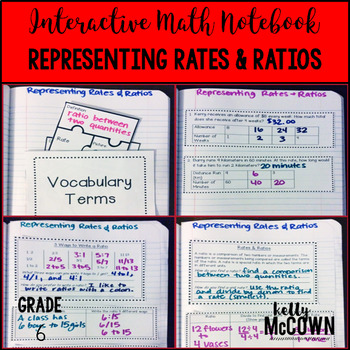These interactive notebook activities are intended to help students understand the concept of a ratio, use ratio language to describe a relationship between two quantities, understand the concept of a unit rate, use rate language to describe a ratio relationship, and use ratio and rate reasoning to solve real-world and mathematical problems.Included are:
-4 different Rates & Ratios activities to engage students
-Teacher facilitated activity for 60-90 minutes of classroom time
-Activities include: Vocabulary, Practice of Writing Ratio Tables, Reading Ratio Word Problems and Writing Ratios 3 Different Ways, and Finding Unit Rates
-Examples of Completed Interactive Math Notebook Activities are includedThese interactive notebook activities are intended to help students understand how to use ratio and rate reasoning to solve real-world and mathematical problems. Students will learn how to reason about tables of equivalent ratios, use double number line diagrams and equations..

Included are:
-4 different Rates & Ratios activities to engage students
-Teacher facilitated activity for 60-90 minutes of classroom time
-Activities include: Vocabulary, Practice of Writing Equivalent Ratios, Finding Unit Rates for different quantities, and Finding Unit Rate Prices of sales items.
-Examples of Completed Interactive Math Notebook Activities are includedThis math station activity is intended to help students understand the concept of a ratio, use ratio language to describe a relationship between two quantities, understand the concept of a unit rate, use rate language to describe a ratio relationship, and use ratio and rate reasoning to solve real-world and mathematical problems.Included are:
-6 different stations to engage students
-Teacher facilitated activity for 60-90 minutes of classroom time
-Stations include:
1. Vocabulary
3. Practice of Rates & Ratios
4. Additional Practice of Rates & Ratios
5. Real World applications of Ratios
6. Word problems of Rates & Ratios
-Student Station Guide helps students record their answers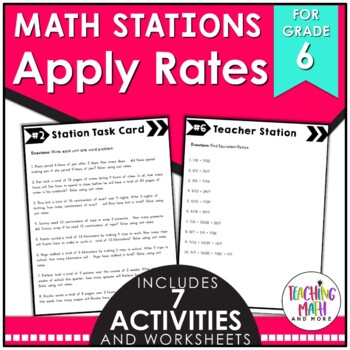This math station activity is intended to help students understand how to use ratio and rate reasoning to solve real-world and mathematical problems. Students will learn how to reason about tables of equivalent ratios, use double number line diagrams and equations.

Included are:
-6 different stations to engage students
-Teacher facilitated activity for 60-90 minutes of classroom time
-Stations include:
1. Vocabulary
3. Practice of Rates & Ratios
4. Additional Practice of Rates & Ratios
5. Real World applications of Ratios
6. Word problems of Rates & Ratios
-Student Station Guide helps students record their answers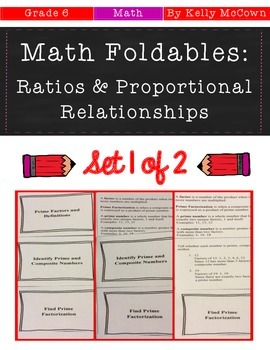These math foldables are for students to be able to understand the concept of a ratio, use ratio language, understand the concept of a unit rate, use ratio and rate reasoning to solve real-world and mathematical problems, make tables of equivalent ratios, and solve unit rate problems.

Included are:
-6 different NO PREP Math Foldables to engage students in note taking
-Teacher facilitated activity for 15-20 minutes of classroom time each
-PRINT & GO teach foldables that are ready with NO PREP
-Directions for using Math Foldables in your classroom
-Options for scaffolding with blank templates
-Foldables included:
Foldable: Ratios & Rates
Foldable: Ratio Tables
Foldable: Proportions
Foldable: Solving Proportions
Foldable: Sequence and Expressions
Foldable: Proportions and Equations
Foldable: Four Blank TemplatesThis November Math NO PREP packet that will keep your sixth graders engaged! This packet is just plain fun. Not only is it PACKED with sixth-grade common core math problems, it also gives students fun coloring, puzzles, and problem solving. Use this packet for bellwork, classwork, extra credit, fast finishers, or homework!

Topics Covered:
-Operations with Fractions
-Graphing on a Coordinate Plane
-Operations with Decimals
-Represent Ratios & Rates
-Apply Ratios & Rates
I hope these resources are helpful in getting your students engaged in Rates & Ratios. Keeping students actively engaged in the learning process will ensure that they are in charge of their own learning. The goal of learning Rates and Ratios is for students to develop a foundational knowledge to continue to grow upon in seventh grade.

## Wednesday, October 19, 2016

### Building a Math Classroom Word Wall {Grades 3 to 8}

I have seen many pretty classrooms. Neat, tidy, bright, colorful, and all teacher made. Do the students benefit from a classroom environment that they create together with the teacher or one that is purely teacher made?

I have found that students take great delight in creating the classroom environment with their classmates. They love to see their student work displayed where THEY put it. They love to see and MAKE sense of how the classroom is decorated and put together.

For the past ten years I have used Word Walls in my Math classroom and the best technique I found was not buying one already made, but having the students create it themselves and FOR THEMSELVES. This simple technique allowed them to take ownership of the Math vocabulary and build upon it through their own learning process.

Before {Empty Wall}

After {Close-up of Math Word Wall}

Create your own word wall in your math classroom! No Prep vocabulary cards are ready for you! Just print and go. There are two versions:

These are great for students to build upon past vocabulary and continue to grow and learn concepts on their own.

This was a very important resource in my classroom. I used it every day! I referred to it daily, had my students actively engaged in building the word wall, and the best part was it was SOOO easy to do. I hope this resource becomes a very helpful tool for your students too!

Happy Teaching!

## Monday, October 17, 2016

### Teaching Writing Linear Equations {Grade 8}

One of my favorite topics to teach in eighth grade math {Pre-Algebra} was teaching students how to write Linear Equations! Students have prior knowledge of numbers, expressions, and variables which helps them to be able to write equations that are linear.

I have listed here four activities to review and practice Writing Linear Equations. I hope these help jump start your lesson planning into Linear Equations. The key to getting your students involved in the learning process is to include interactive, visual, engaging activities for them to actively participate in.These interactive notebook activities are intended to help students understand how to construct a function to model a linear relationship, construct and interpret scatter plots for bivariate data, investigate patterns of association between two quantities, know that straight lines are widely used to model relationships between two variables, and use the equation of a linear model to solve problems in the context of bivariate data.

Included are:
-4 different Writing Linear Equations activities to engage students
-Teacher facilitated activity for 60-90 minutes of classroom time
-Activities include: Vocabulary, Writing a rule (linear equation) from a graph, Writing a rule from two points using slope-intercept form, and finding the slope with the slope formula.
-Examples of Completed Interactive Math Notebook Activities are includedThis math station activity is intended to help students understand how to construct a function to model a linear relationship, construct and interpret scatter plots for bivariate data, investigate patterns of association between two quantities, know that straight lines are widely used to model relationships between two variables, and use the equation of a linear model to solve problems in the context of bivariate data.

Included are:
-6 different stations to engage students
-Teacher facilitated activity for 60-90 minutes of classroom time
-Stations include:
1. Vocabulary
3. Practice of Writing Linear Equations
4. Additional Practice of Writing Linear Equations
5. Real World applications of Writing Linear Equations
6. Word problems of Writing Linear Equations
-Student Station Guide helps students record their answersThis lesson unit is intended to help you assess how well students are able to understand how to model a linear relationship between two quantities and determine the rate of change (slope) from two (x,y) values.Included in this lesson are:
-card sort activity with concept development of linear equations
-one extension activity for students to deepen their conception of linear equations
-answer keys for all the assessments and activities
-how the lesson is tied to common core and mathematical practicesThis October Math NO PREP packet that will keep your eighth graders engaged! This packet is just plain fun. Not only is it PACKED with seventh-grade common core math problems, it also gives students fun coloring, puzzles, and problem solving. Use this packet for bellwork, classwork, extra credit, fast finishers, or homework!

CLICK HERE TO WATCH THE VIDEO TUTORIAL OF THE NO PREP MATH PACKETS FOR OCTOBER

Have fun with Writing Linear Equations! Find a creative approach and teach it. October is a long and hard time to engage your students. YET IT IS the perfect time to engage your students in Math and the rewards will be higher in the end of year!

## Friday, October 14, 2016

### Math Morning Work: 3rd Grade

This is Detective work for only the keenest of eye! Students will be looking for clues to solve each day's problem. They must analyze and figure out what the student did mathematically and whether or not there must be corrections. This is a Morning Math Set for the 1st Nine Weeks covering the benchmarks {Common Core State Standards 3.MD.B.33.MD.B.43.NBT.A.1,3.NBT.A.23.OA.A.13.OA.A.3}.

This Morning Math Work: Daily Detective Math Work Packet for 3rd Graders is for daily math practice and review. It is Common Core standards-based and sequenced in an order to review ALL CCSS for third grade. These problems can be used as Math Morning Work, Math Centers Work, Homework, Classwork, or for Early Finishers!!

Table of Contents clearly displays what the contents of the Math Morning Work Packet for Quarter 1 contains. There are directions for teachers on how to get started. TIPS for the Detectives {students} on how to solve error analysis problems and look for mistakes. Super Detectives are students who have successfully completed all 9 Case Loads {9 weeks of work} and earn recognition!

Included in this packet are:
-9 weeks of Review & Error Analysis of Math Skills
-9 Different Detective "Case Loads" for students to solve

-HIGH QUALITY Detective Clip Art used

Topics Covered are:
-Compatible Numbers
-Rounding
-Frequency Tables
-Pictographs
-Bar Graphs
-Line Plots
-Multiplication
-Multiplication Arrays

## Wednesday, October 12, 2016

### Middle School Interactive Math Notebook {Grade 6}

Do you use Interactive Math Notebooks? This is an engaging Interactive Math Notebook Bundle for Grade 6. Every Common Core State Standard is addressed in this Bundle. It is very versatile and easy to use for any student.

This Middle School Interactive Math Notebook Bundle is a COMPLETE BUNDLE covering ALL 6th grade Common Core Standards! This Bundle is 20% OFF the original price of all total interactive math notebook activities.

Each Interactive Math Notebook Activity includes 4 different activities {vocabulary, notes, practice, and applications} for that skill set addressed. These activities are easily teacher facilitated {as the teacher you give the instruction and the students then cut, glue, paste, and engage in the activities on their own with limited guiding}. You can use 1 or all 4 of the activities included to meet the needs of your students. Also included is the Middle School Interactive Math Notebooks 101 Guide. {Click HERE to PREVIEW}.

There are over 300 PAGES of Interactive Math Notebook Activities for students to be actively participating in Mathematics with. All of the activities have students engaging in the learning process and making learning gains for themselves. These activities are rigorous and add depth to any Grade 6 Mathematics Classroom. As always, these are activities that I have used in the classroom and only share them with you because they WORK, are EFFECTIVE, and INCREASE student learning gains! Check out the Bundle HERE to see what Interactive Math Notebooks could do for your students.

## Monday, October 3, 2016

### Teaching Rates & Proportionality {Grade 7}

I LOVE teaching proportions, ratios, and rates! It's my favorite time of year when everything is equivalent and proportional. With a love for this topic I have created resources to help develop conceptual knowledge of rates and rigorous activities for practicing proportions. Here are some of my favorites!This Interactive Math Notebook activity is intended to help students understand how to compute unit rates and ratios, recognize and represent proportional relationships between quantities, decide whether two quantities are proportional, identify the unit rate, represent proportional relationships, and use proportional relationships to solve multistep ratio problems.Included are:
-4 different Rates & Proportions activities to engage students
-Teacher facilitated activity for 60-90 minutes of classroom time
-Activities include: Vocabulary, finding missing numbers and solving proportions, how to find and name equivalent ratios, and how to find and solve unit rate problems.
-Examples of Completed Interactive Math Notebook Activities are included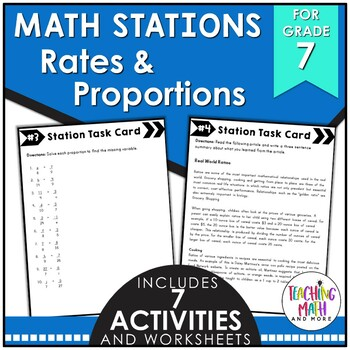This math station activity is intended to help students understand how to compute unit rates and ratios, recognize and represent proportional relationships between quantities, decide whether two quantities are proportional, identify the unit rate, represent proportional relationships, and use proportional relationships to solve multistep ratio problems.Included are:
-6 different stations to engage students
-Teacher facilitated activity for 60-90 minutes of classroom time
-Stations include:
1. Vocabulary
3. Practice of Rates & Proportionality
4. Additional Practice of Rates & Proportionality
5. Real World applications of Rates & Proportionality
6. Word problems of Rates & Proportionality
-Student Station Guide helps students record their answers{Click HERE for Unit Rates & Ratios Card Sort Activity & Assessment}
This lesson unit is intended to help you assess how well students are able to compute unit rates as they are associated with ratios of fractions, lengths, areas, and other quantities measured in different units.Included in this lesson are:
-card sort activity with concept development of ratios and unit rates
-one extension activity for students to deepen their conception of unit rates
-answer keys for all the assessments and activities
-how the lesson is tied to common core and mathematical practicesThis lesson unit is intended to help you assess how well students are able to solve problems involving scale drawings of geometric figures, including computing actual lengths and areas from a scale drawing and reproducing a scale drawing at a different scale.
Included in this lesson are:
-card sort activity with concept development of scale drawings
-one extension activity for students to deepen their conception of scale drawings
-answer keys for all the assessments and activities
-how the lesson is tied to common core and mathematical practicesThese math foldable notes are for students to be able to understand compute unit rates, ratios with fractions, measure quantities in like or different units, recognize and represent proportional relationships, decide whether two quantities are proportional, identify the constant of proportionality (unit rate), show the unit rate in tables, graphs and equations, represent proportional relationships by equations, explain points on a graph of a proportional relationship, and use proportional relationships to solve multistep ratio and percent problems.
Included are:
-7 different NO PREP Math Foldable Notes to engage students in note taking
-Teacher facilitated activity for 15-20 minutes of classroom time each
-PRINT & GO foldable notes that are ready with NO PREP
-Directions for using Math Foldable Notes in your classroom
-Options for scaffolding with blank templates
-Foldables included:
Foldable: Ratios
Foldable: Rates & Unit Rates
Foldable: Rate of Change & Slope
Foldable: Measurement Changing Units
Foldable: Solving Proportions
Foldable: Scale Drawings
Foldable: Fractions, Decimals, & Percents
Foldable: Four Blank Templates

There are lots of different approaches to teaching rates and proportions. I have found that interactive tools help the most. By using Card Sort activities you can guide and facilitate the learning process for mastery of concepts. When you find the best way to reach your students, your learning gains will increase the most!